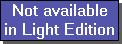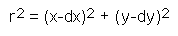The SDL Component Suite is an industry leading collection of components supporting scientific and engineering computing. Please visit the SDL Web site for more information....## CalcCircleFitUnit: SDL_math2 Class: Declaration: procedure CalcCircleFit (var r, dx, dy: double);

The procedure CalcCircleFit calculates the best fitting circle for a given set of data. The circle is determined by the equationThe values of x and y are given by the data samples, the parameters dx, dy, and r are estimated by CalcCircleFit using a least squares approximation.

The data points [x,y] have to be entered using the routine EnterStatValue. A minimum number of 3 values is required in order to apply CalcCircleFit. Do not forget to reset the statistics calculation before entering any new data sets (use Init)

 Example: This method is used in the following example program (see http://www.lohninger.com/examples.html for downloading the code): curvefit

Last Update: 2012-Oct-20